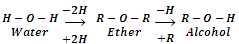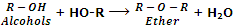What are Ethers and its types?

Ethers are the compounds with general formula or CnH2n+2O (same as monohydric alcohols). These are symbolized through a general structure. R-O-R'. They may be regarded as dialkyl derivatives of water or mono alkyl derivatives of alcohols.They are also considered as anhydrides of alcohols because they can be obtained by the elimination of water molecule from two alcohol molecules.The groups R and R' in ether may either be same or different. In case these groups are same, the compounds are known as simple ethers or symmetrical ethers. On the other hand, if R and R' groups are different, the compounds are called mixed ether or unsymmetrical ethers.

C2H5-O-C2H5      Simple ether

C2H5-O-CH3         Mixed ether

Ethers may also be classified into two categories as aliphatic ethers and aromatic ethers. In aliphatic ethers, the groups R and R' are both alkyl groups whereas in aromatic ethers, one or both R and R' groups are aryl groups. Ether with one alkyl and one aryl groups are also called aryl alkyl ethers or phenolic ethers whereas ethers with both aryl groups are called diaryl ethers. Few examples are taken below:#### Related Questions in Chemistry

• ##### Q :Mole fraction of water and ethanol Give

Give me answer of this question. A solution contains 1 mole of water and 4 mole of ethanol. The mole fraction of water and ethanol will be: (a) 0.2 water + 0.8 ethanol (b) 0.4 water + 0.6 ethanol (c) 0.6 water + 0.8 ethanol (d) 0.8 water + 0.2 ethanol

• ##### Q :Wavelengths which the human eye can see

Briefly state the wavelengths which the human eye can see?

• ##### Q :Question based on vapour pressure and

Give me answer of this question. The vapour pressure of water at 20degreeC is 17.54 mm. When 20g of a non-ionic, substance is dissolved in 100g of water, the vapour pressure is lowered by 0.30 mm. What is the molecular weight of the substances: (a) 210.2 (b) 206.88

• ##### Q :Osmotic Pressure The O.P. (Osmotic

The O.P. (Osmotic Pressure) of equimolar solution of Urea, BaCl2 and AlCl3, will be in the order:
(a) AlCl3 > BaCl2 > Urea  (b) BaCl2 > AlCl3 > Urea  (c) Urea > BaCl2<

• ##### Q :Oxoacids of halogens Why oxidising

Why oxidising character of oxoacids of halogens decreases as oxidation number increases?

• ##### Q :Neutralisation of phosphorous acids

Provide solution of this question. To neutralise completely 20 mL of 0.1 M aqueous solution of phosphorous acid (H3 PO3) the volume of 0.1 M aqueous KOH solution required is: (a) 40 mL (b) 20 mL (c) 10 mL (d) 60 mL

• ##### Q :Question based on lowest vapour pressure

Give me answer of this question. Among the following substances the lowest vapour pressure is exerted by: (a) Water (b) Mercury (c) Kerosene (d) Rectified spirit

• ##### Q :Vapour pressure Vapour pressure of

Vapour pressure of methanol in water Give me answer of this question. An aqueous solution of methanol in water has vapour pressure: (a) Equal to that of water (b) Equal to that of methanol (c) More than that of water (d) Less than that of water

• ##### Q :Molal concentration Select the right

Select the right answer of the question. If one mole of any substance is present in of solvent, then: (a) It shows molar concentration (b) It shows molal concentration (c) It shows normality (d) It shows strength.

• ##### Q :Determining highest normality What is

What is the correct answer. Which of the given solutions contains highest normality: (i) 8 gm of KOH/litre (ii) N phosphoric acid (iii) 6 gm of NaOH /100 ml (iv) 0.5M H2SO4